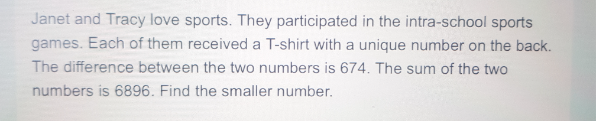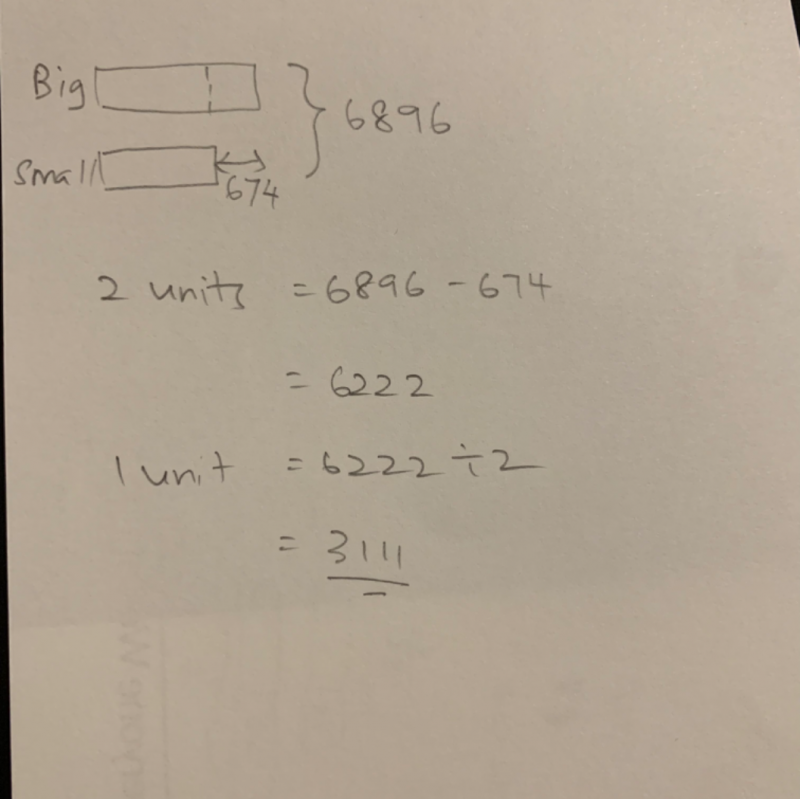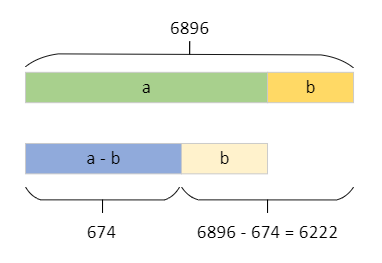# QuestionFor Primary School Maths, we try as much to use models as possible. There are many methods that are used but it’s beyond the child’s level. For example, simultaneous equations and the widely used parts and units methods. It involves some algebra in it. 😊

0 Replies 1 Like

Let the numbers be a and b, where a is the larger one. So we can draw such a block diagram.The top block represents a + b = 6896. The bottom blue bar represents a – b = 674. From the diagram, we see that the difference 6896 – 674 = 6222 is exactly two “b”.

So the smaller number b is 6222/2 = 3111.

Check: b = 3111. So a = 6896 – b = 3785. And a – b = 3785 – 3111 = 674.

I’ve used block diagrams since this is a P3 problem. But if you know algebra, then we can write:

a + b = 6896
a –  b = 674

Subtract 2nd equation from 1st equation to get:

(a + b) – (a – b) = 2b = 6896 – 674 = 6222.

So b = 3111.

2 Replies 2 Likes